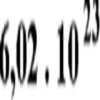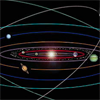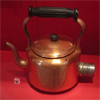# Equations and Formulae

This collection of STEM resources is based around equations and formulae.  Resources are listed in order of stage.### Temperature

##### Age 11 to 14 Challenge Level:

Is there a temperature at which Celsius and Fahrenheit readings are the same?### Back Fitter

##### Age 14 to 16 Challenge Level:

10 graphs of experimental data are given. Can you use a spreadsheet to find algebraic graphs which match them closely, and thus discover the formulae most likely to govern the underlying processes?### Constantly Changing

##### Age 14 to 16 Challenge Level:

Many physical constants are only known to a certain accuracy. Explore the numerical error bounds in the mass of water and its constituents.### How Do You React?

##### Age 14 to 16 Challenge Level:

To investigate the relationship between the distance the ruler drops and the time taken, we need to do some mathematical modelling...### Construct the Solar System

##### Age 14 to 18 Challenge Level:

Make an accurate diagram of the solar system and explore the concept of a grand conjunction.### Electric Kettle

##### Age 14 to 16 Challenge Level:

Explore the relationship between resistance and temperature